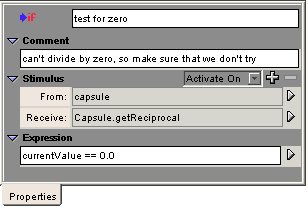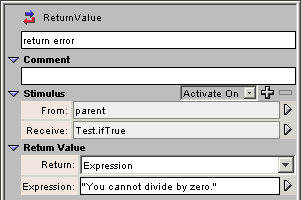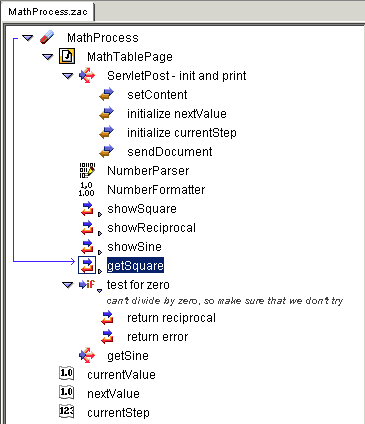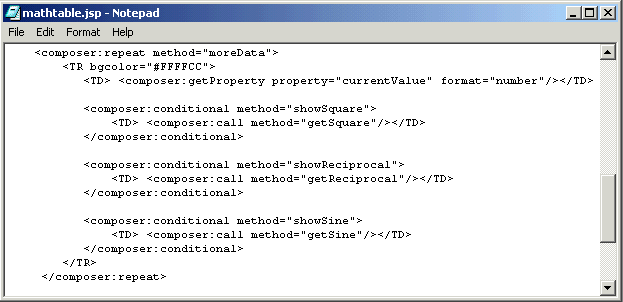# Defining More Complex Behaviors

The getSquare method was a very simple operation to define. The other two operations require more complex behaviors to implement them. A reciprocal is a fraction with one over the number. For instance, the reciprocal of 5 is 1/5. Zero presents a special case for reciprocals. Because you cannot divide by zero in mathematics, you need to provide a way to check that zero is not the value you are operating on, and provide a way for the application to handle that if it does occur. A type of behavior useful for this situation is an IfTest.

Another new behavior type you will create is a script. A script behavior is simply a collection of Java statements. This type of behavior gives developers with some Java knowledge great flexibility. You will create a script that performs the complex mathematical function of calculating a number's sine, and sends the output of that calculation to a variable.

## Error Checking: About IfTests

An IfTest is a behavior type that evaluates an expression and generates one of two different events in response. In the behavior, you specify an expression that you want to evaluate as being either true or false. If the test evaluates as true, an IfTest generates an ifTrue event. If the test expression evaluates as false, it generates an ifFalse event. You use these events to activate behaviors that handle each outcome. IfTests are similar to behaviors that have conditional activation (which you will work with in the next section of the tutorial), but are a little more convenient when you need to respond to either outcome of the test, rather than just a positive outcome.

In this case, for the reciprocal, you need to check whether the current value is 0. If it is not, you want to calculate and display the reciprocal of the value. If it is, you want to print an appropriate message that warns the user, instead of attempting an operation that will fail.

#### To define getReciprocal:

1. Select MathTablePage in the outline.
2. Insert an IfTest behavior from the Palette.
Behaviors -> IfTest
3. Name the IfTest test for zero.
4. Edit the behavior to match the following settings:
• From: capsule
• Receive: Capsule -> New Method... -> getReciprocal
In the New Method dialog, enter `getReciprocal` as the name to Activate On, set the return type to `String`, and click `OK`. No argument is needed for this method.
• Expression: currentValue == 0.0

Make sure you use two equals signs. This notation compares whether currentValue and 0.0 are the same, rather than assigning a value of 0.0 to currentValue, as one equals sign would do.Now that you have set up the IfTest, you can add behaviors to it to respond to the ifFalse and ifTrue events that it generates. You want to calculate the reciprocal if the current value does not equal zero, and print an error message if it does equal zero.

#### To create return reciprocal:

1. Make sure test for zero is selected in the outline.
2. Insert a new ReturnValue behavior as a child of test for zero.
Insert -> Other Behaviors -> ReturnValue
3. Name the new behavior return reciprocal.
4. Edit the return reciprocal behavior to match the following settings:
• From: parent
• Receive: Test -> ifFalse
• Return: Expression
• Expression: NumberFormatter.format(1.0 / currentValue)This behavior divides one by the current value and returns the value as a string to the JSP.

#### To create return error:

1. Select test for zero in the outline.
2. Insert a new ReturnValue behavior as a child of test for zero.
Insert -> Other Behaviors -> ReturnValue
3. Name the new behavior return error.
4. Edit return error's behavior to match the following settings:
• From: parent
• Receive: Test -> ifTrue
• Return: Expression
• Expression: "You cannot divide by 0."Instead of calculating a reciprocal, this behavior returns an error String to the capsule.

## About Scripts

AppComposer's script behavior type is a feature that allows more advanced Java users to quickly implement a piece of functionality using a familiar technique. Ideally, you should be able to acquire or design components that provide the functionality you need without having to use scripts to tie them together. Scripts can execute any series of Java statements. The statements must each have correct syntax, but you do not need to worry about creating classes for them. AppComposer takes care of that for you.

To calculate and print the sines of your series of numbers, you need to create an action group that contains: a script to do the calculation, a variable to hold the result, and a behavior that formats the result as a string so that the JSP file can use it.

#### To define getSine:

1. Select MathTablePage in the outline.
2. Insert an action group behavior as a child of MathTablePage.
Behaviors -> ActionGroup
3. Name the action group getSine.
4. Edit getSine's activation event to match the following settings:
• From: capsule
• Received: Capsule -> New Method -> getSine
In the New Method dialog, enter `getSine` as the name to Activate On, set the return type to `String`, and click `OK`.Now you need to add the behaviors that make up the getSine action group, and a variable that these behaviors use.

#### To add a variable for sine:

1. Select getSine in the outline.
2. Insert a double data item as a child of getSine.
Data Items -> double
3. Name the double sine.

Doubles are a data type that contains a decimal.

#### To calculate the sine using a script:

1. Select getSine in the outline.
2. Insert a script behavior as a child of getSine.
Behaviors -> Script
3. Name the script calculate sine.
4. Edit the script to match the following settings:
• ```Script
double radians = currentValue * java.lang.Math.PI/360.0;
sine = java.lang.Math.sin(radians);```Script behaviors can execute any Java code. This code creates a variable called radians and uses it to store the result from (currentValue * PI) /360.0. The script then uses that result to calculate the sine of currentValue, and stores the result in the variable called sine. The value of PI is called from Java's Math class, which stores many mathematical constants and functions so you do not have to create them in each class that uses them.

The action group still needs a way to send out the result of this calculation in a format the JSP can use.

#### To return the sine:

1. Select getSine in the outline.
2. Insert a new ReturnValue behavior as a child of getSine.
Insert -> Other Behaviors -> ReturnValue
3. Name the behavior return sine string.
4. Edit return sine string to match the following settings:
• Send To: capsule
• Return: Expression
• Expression: NumberFormatter.format(sine)This behavior turns the numeric value in the sine variable into a String and returns it to the JSP.

Your capsule should now look like this:If you try to run it, you still get output of only the header row in the table, even though you have now defined the behaviors you need to calculate the operations specified by a user. This is because the JSP file encloses all of the conditional and call tags that fill in the values of the results table within a <composer: repeat > tag.Like the conditional tag, if the servlet does not respond to it, the JSP skips whatever the tag encloses.

In the next section, you will create a loop that activates on the repeat method call from the JSP, and builds the table for the results of the operations.© 2003 DigiSlice Corporation